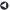### Statistical Services OfferedBack to List of Services

#### Multivariate Statistical Methods

Univariate statistics consider only one response (or dependent) variable at a time. Examples are the sample mean and ANOVA. Multivariate statistics consider more than one response variable at a time. Examples are a vector of sample means and MANOVA. Multivariate statistics encompasses a broad range of statistical methods including:

Multivariate Analysis of Variance (MANOVA) is an extension of the concepts and techniques of ANOVA to situations with multiple dependent variables. A linear statistical model, MANOVA tests for significant differences between groups on two or more related dependent variables simultaneously while accounting for the correlation among the dependent variables. Where ANOVA tests for differences among group means, MANOVA tests for differences among the multivariate centroids of groups. Unlike ANOVA, MANOVA takes into account relationships among the dependent variables as well as the relationship between independent and dependent variables.

Multivariate Analysis of Covariance (MANCOVA) tests for significant differences between groups on a set of related dependent variables while statistically controlling for a linearly related continuous covariate.

Multivariate Multiple Regression tests for a significant linear relationship between a set of predictors and a set of related responses while accounting for the correlations among the responses. It is a type of general linear model and is similar to MANOVA: the hypotheses are similar, the estimates are obtained in a similar way, the assumptions are similar, and the multivariate test statistics are the same.

Canonical Correlation: Suppose you are interested in the relationship between one set of variables and another set of variables without assuming unidirectional causation from one set to the other. Canonical correlation may be suitable for this analysis. Canonical correlation is a generalization of MANOVA, MANCOVA, and multivariate multiple regression. It is useful for a broad range of applications, including handling continuous and categorical variables and evaluating bidirectional associations between sets of variables as well as between an individual variable and a set of variables.

Multivariate Repeated Measures Analysis can be used for analyzing repeated measures data with one response per time point on the same unit, subject, or case. When there are more than one dependent variable measured across several time points, Doubly Multivariate Repeated Measures Analysis (also called multi-response repeated measures) is appropriate. It is referred to as a doubly multivariate method because of multiple time points and multiple dependent variables. The technique tests for significant group differences over time across a set of response variables measured at each time while accounting for the correlation among the responses.

Discriminant Function Analysis (DFA): A major use of DFA is predicting membership in groups. This use is similar to that of logistic regression which models the odds of membership in one group versus another group based on values of predictors. Discriminant analysis is a way of determining which variables predict membership in various groups. It is also a dimension reduction method because many predictor variables are reduced to a smaller number of discriminant functions based on the number of groups that exist in the data.

Although DFA can be viewed as a multivariate generalization of logistic regression, its computational techniques are more similar to those of MANOVA and canonical correlation analysis than to those of logistic regression. Discriminant analysis can also be applied in a stepwise manner to try to find which variables are best for predicting groups; this procedure reduces the number of predictors used to obtain the discriminant functions.

Principal Components Analysis (PCA) is used to reduce many correlated variables to a more manageable number of uncorrelated variables. PCA finds linear combinations of many correlated variables which account for most of the variation in a set of variables with a smaller number of linear combinations of those variables. PCA is commonly used to reduce a large number of collinear (or correlated) predictors to a small number of independent (or orthogonal) predictors for multiple regression analysis. PCA does not assume an underlying latent factor structure.

Factor Analysis bears some superficial resemblance to principal components analysis, but its objective is fundamentally different from that of PCA. Factor Analysis assumes an underlying factor structure and seeks to identify latent variables that explain common variability among input variables. PCA, in contrast, extracts components to explain the total variation in the input variables.

Exploratory Factor Analysis identifies underlying factors while Confirmatory Factor Analysis (CFA) validates them. CFA is often performed by Structural Equation Modeling (SEM) which can be used to investigate relationships among a set of continuous or discrete responses, which can be observed or latent, and a set of continuous or discrete predictors, which can be observed or latent.Back to List of Services